## This means that a length of 1 cm on the photograph above corresponds to a length of 200cm or 2 m, of the actual engine. The scale can also be written as the ratio of two lengths.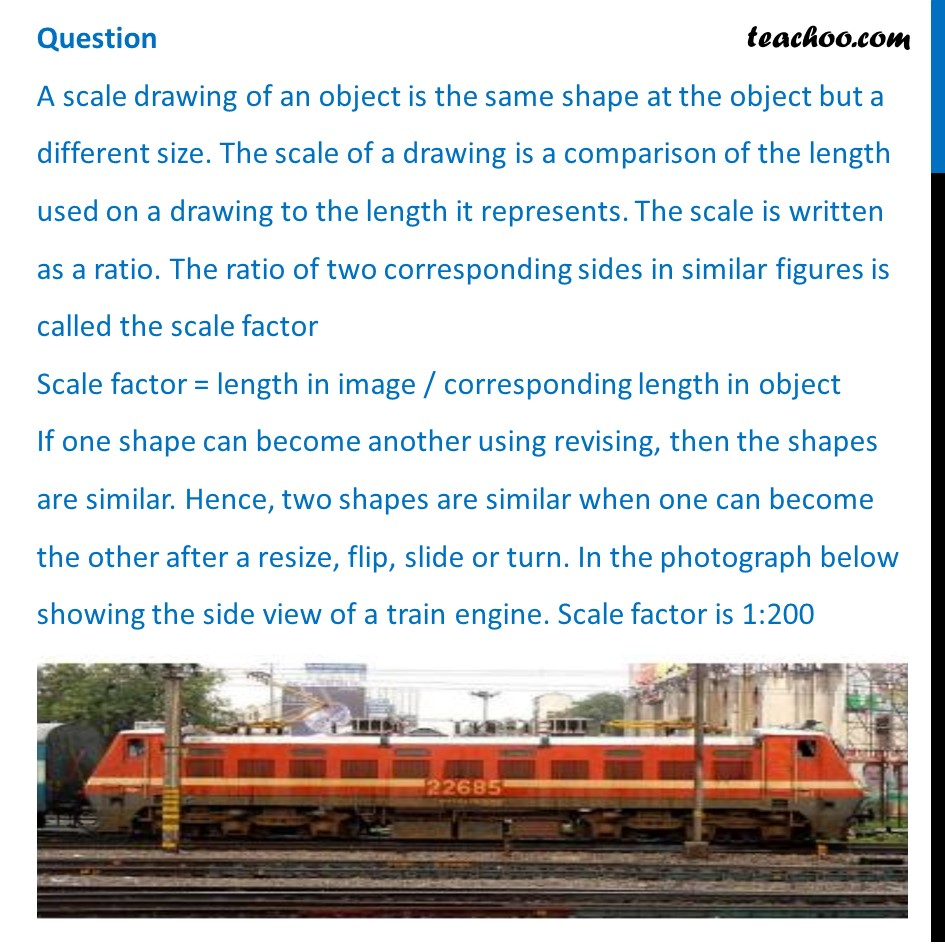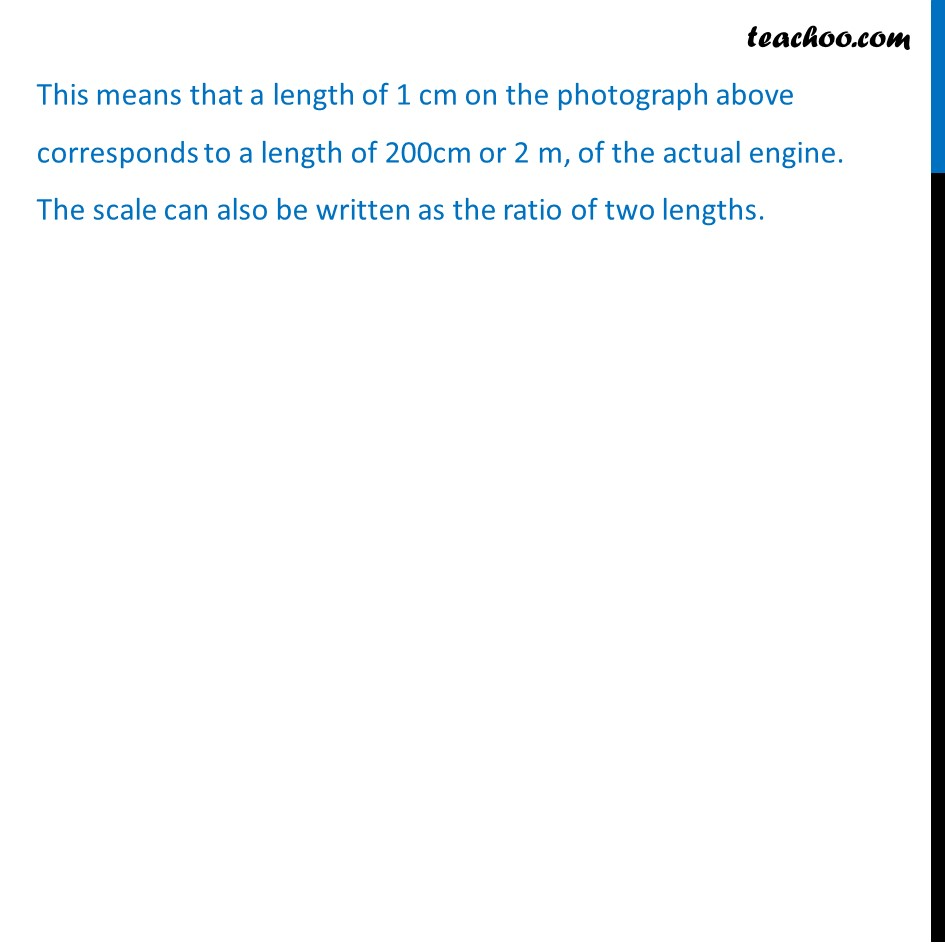## If the length of the model is 11cm, then the overall length of the engine in the photograph above, including the couplings(mechanism used to connect) is: (a) 22 cm (b) 220 cm (c) 220 m (d) 22 m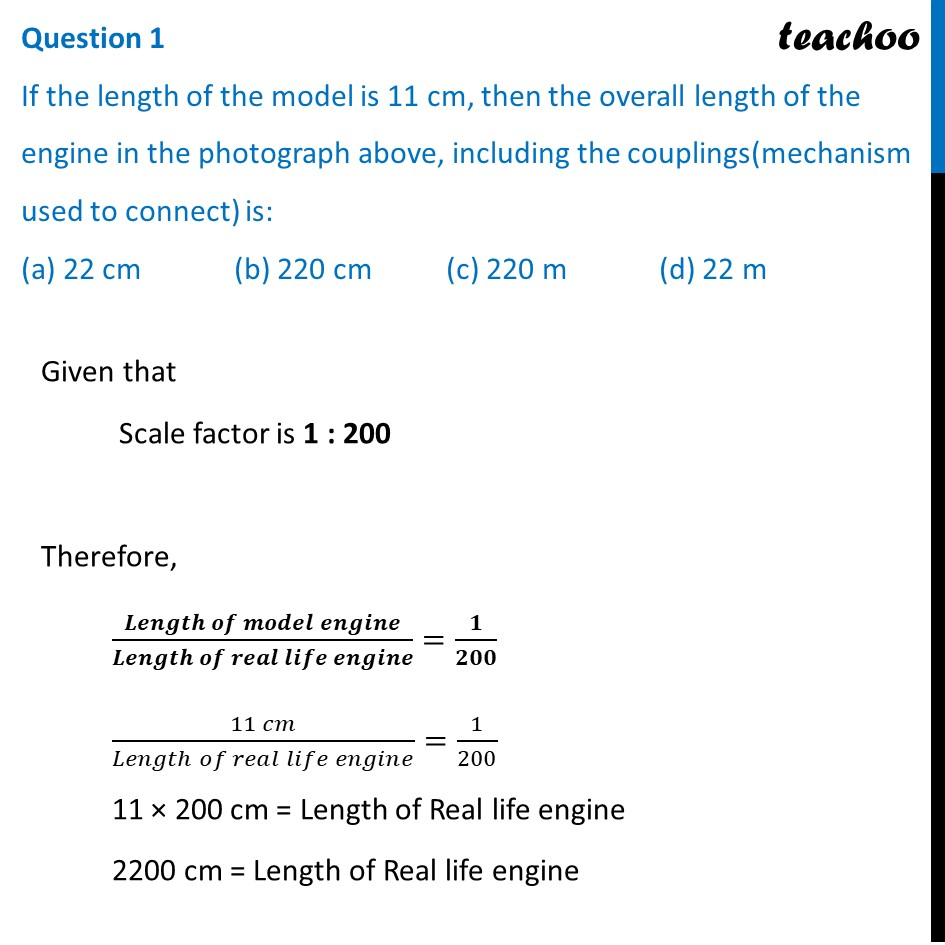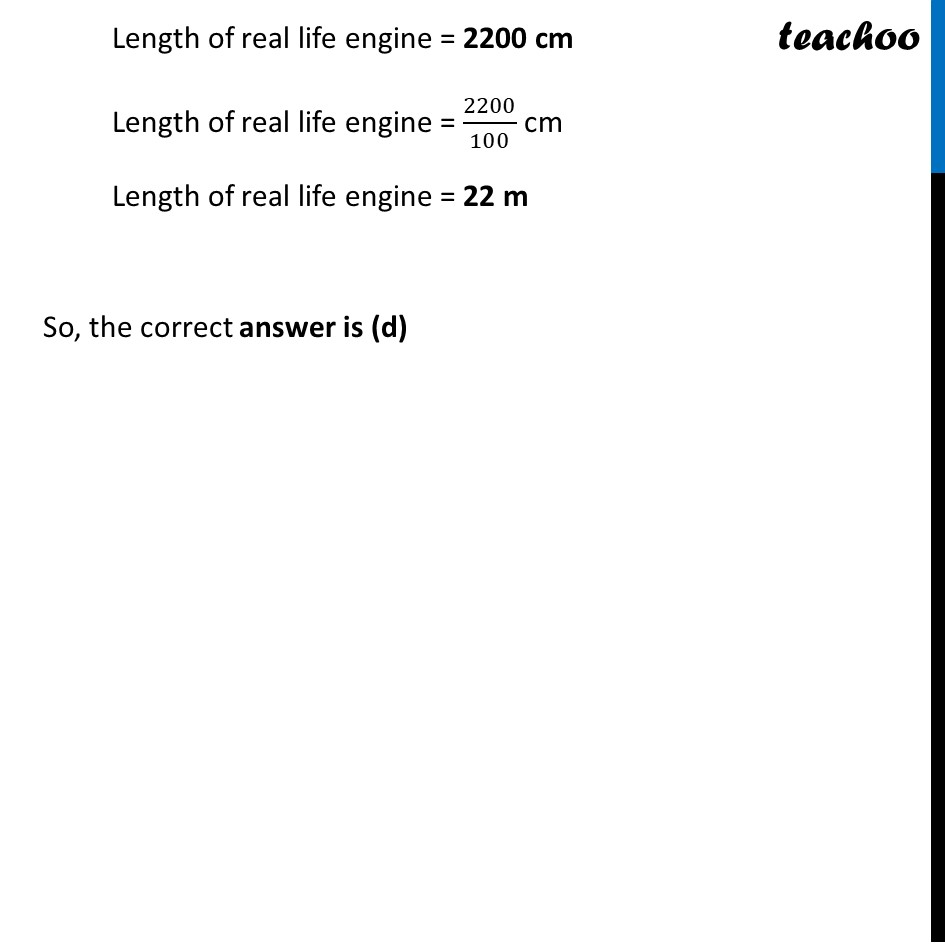## What will affect the similarity of any two polygons? (a) They are flipped horizontally (b) They are dilated by a scale factor (c) They are translated down (d) They are not the mirror image of one another.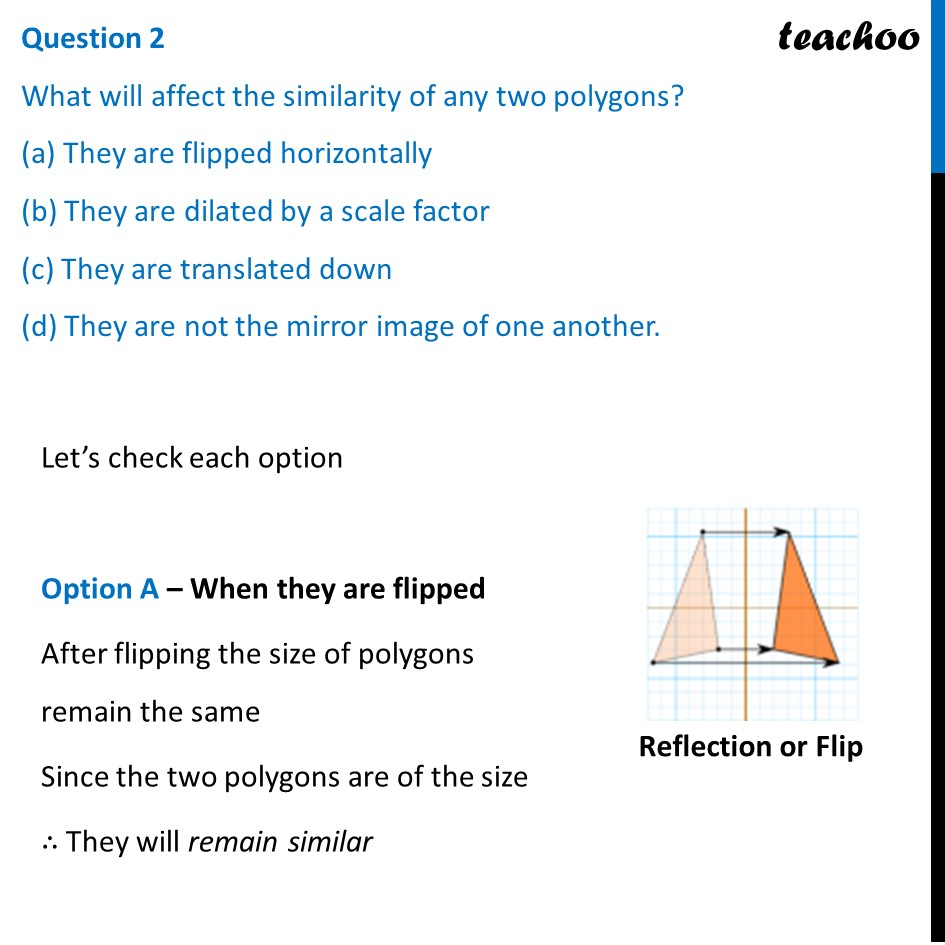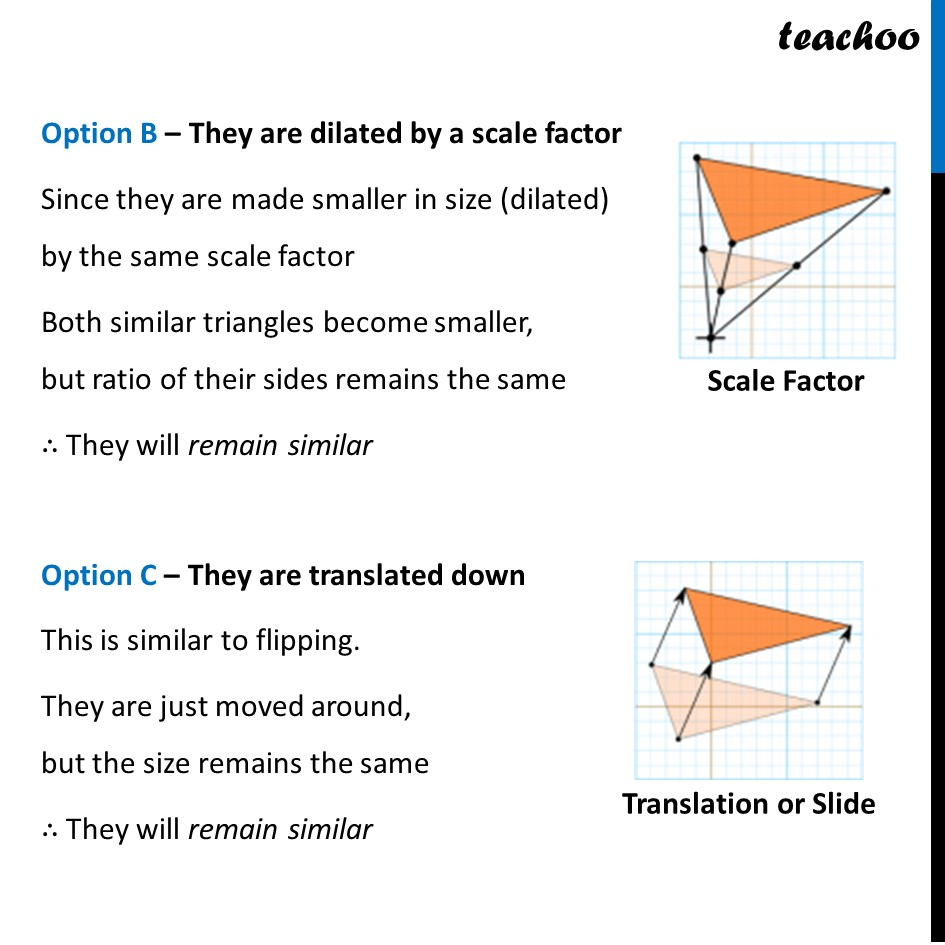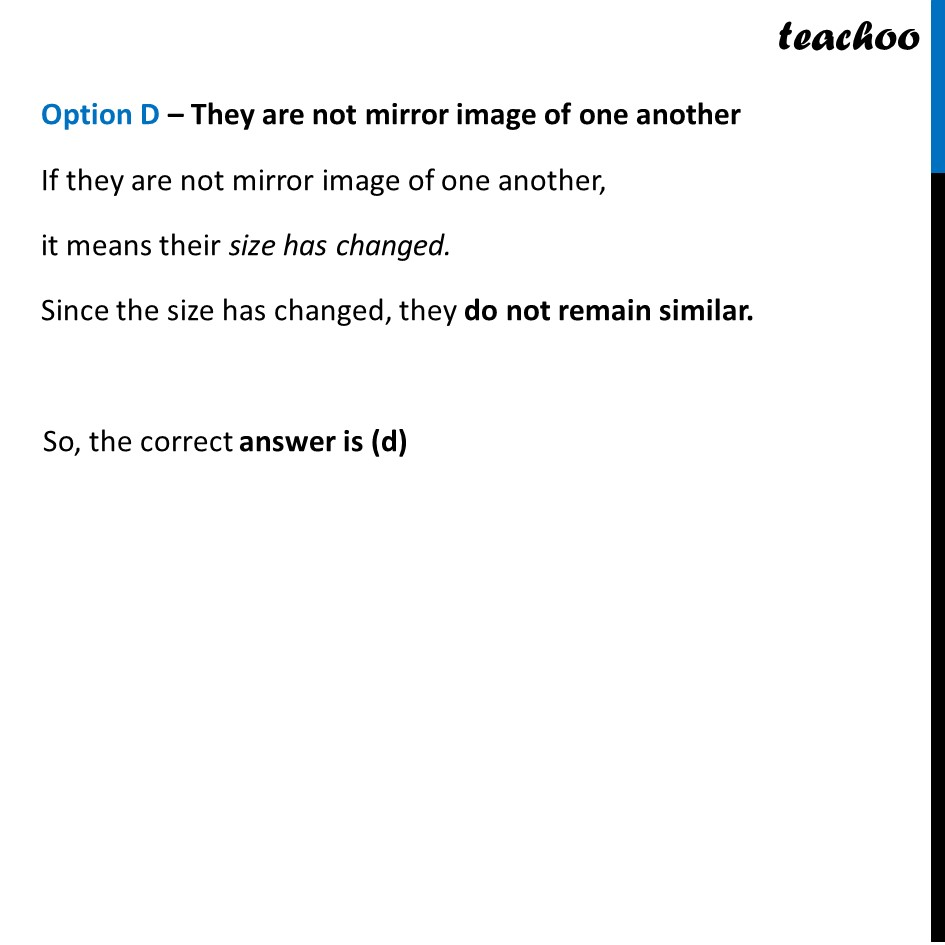## What is the actual width of the door if the width of the door in photograph is 0.35cm? (a) 0.7 m (b) 0.7 cm (c) 0.07 cm (d) 0.07 m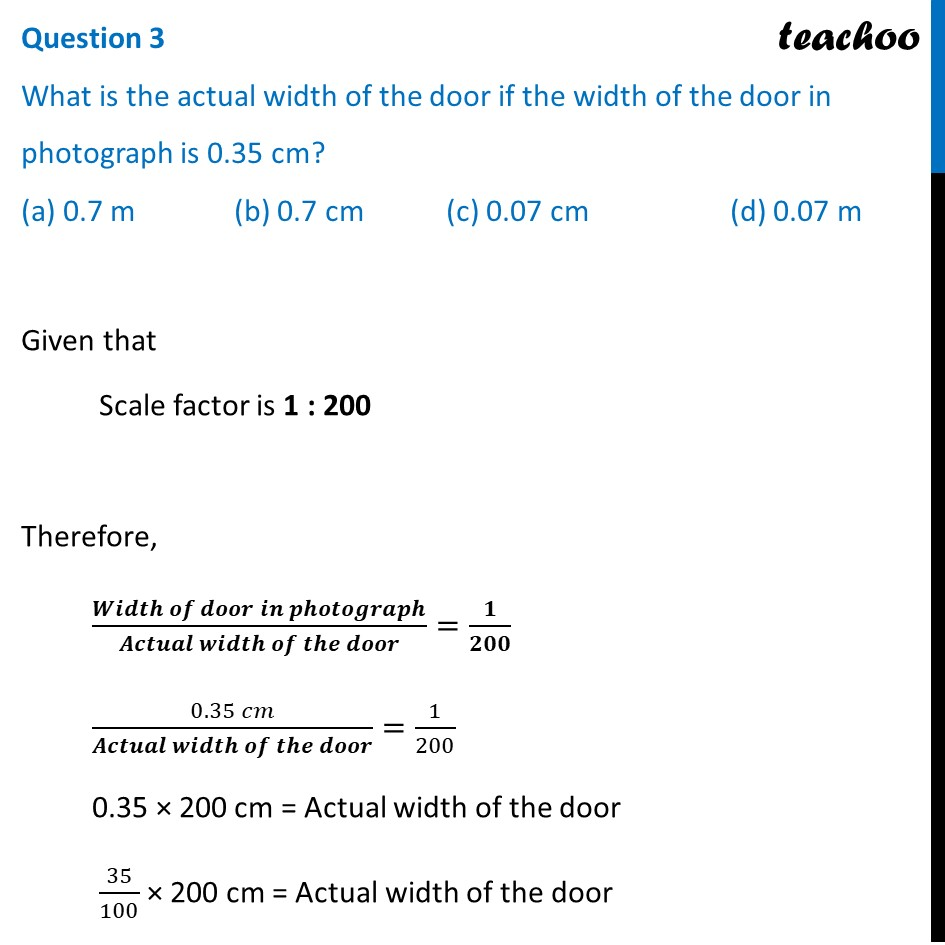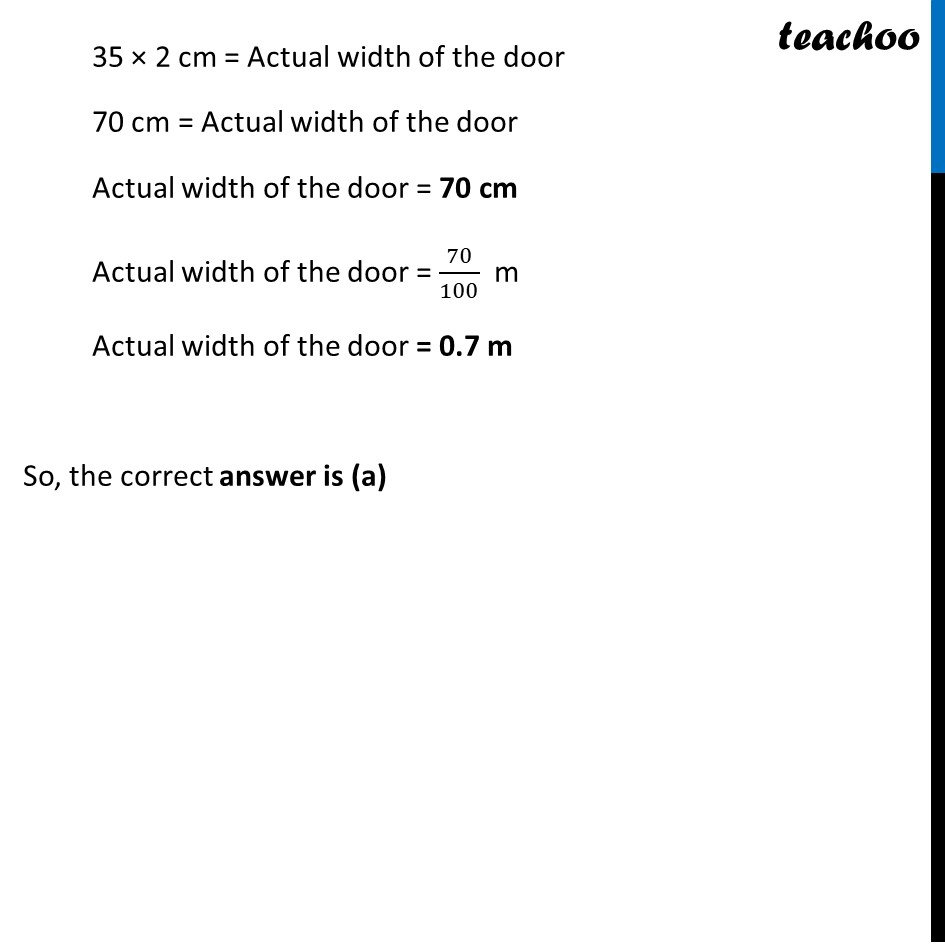## If two similar triangles have a scale factor 5 : 3 which statement regarding the two triangles is true? (a) The ratio of their perimeters is 15 : 1 (b) Their altitudes have a ratio 25 : 15 (c) Their medians have a ratio 10 : 4 (d) Their angle bisectors have a ratio 11 : 5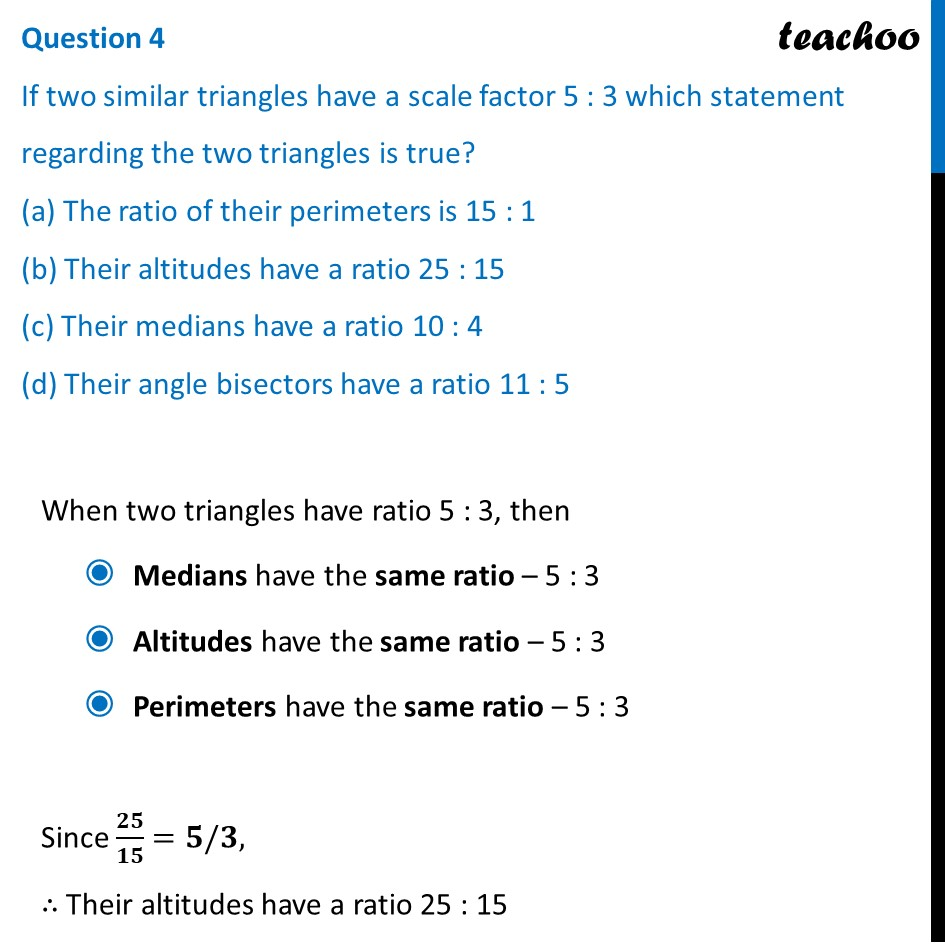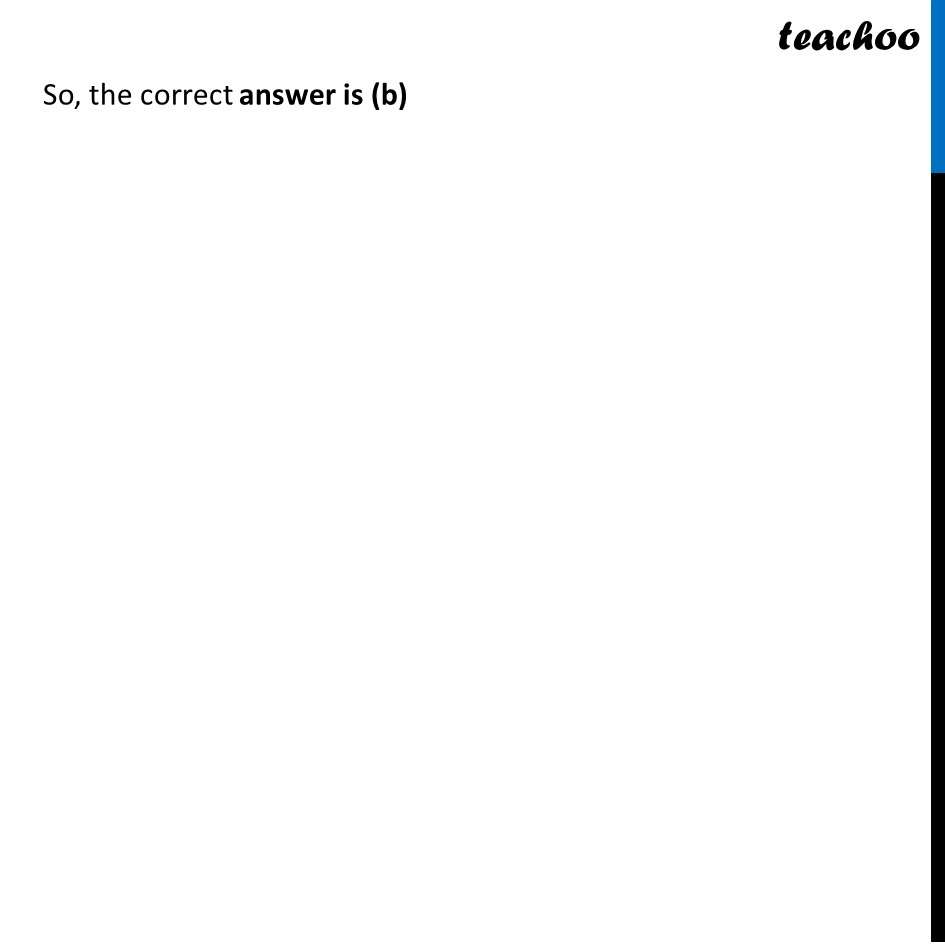## The length of AB in the given figure: (a) 8 cm (b) 6 cm (c) 4 cm (d) 10 cm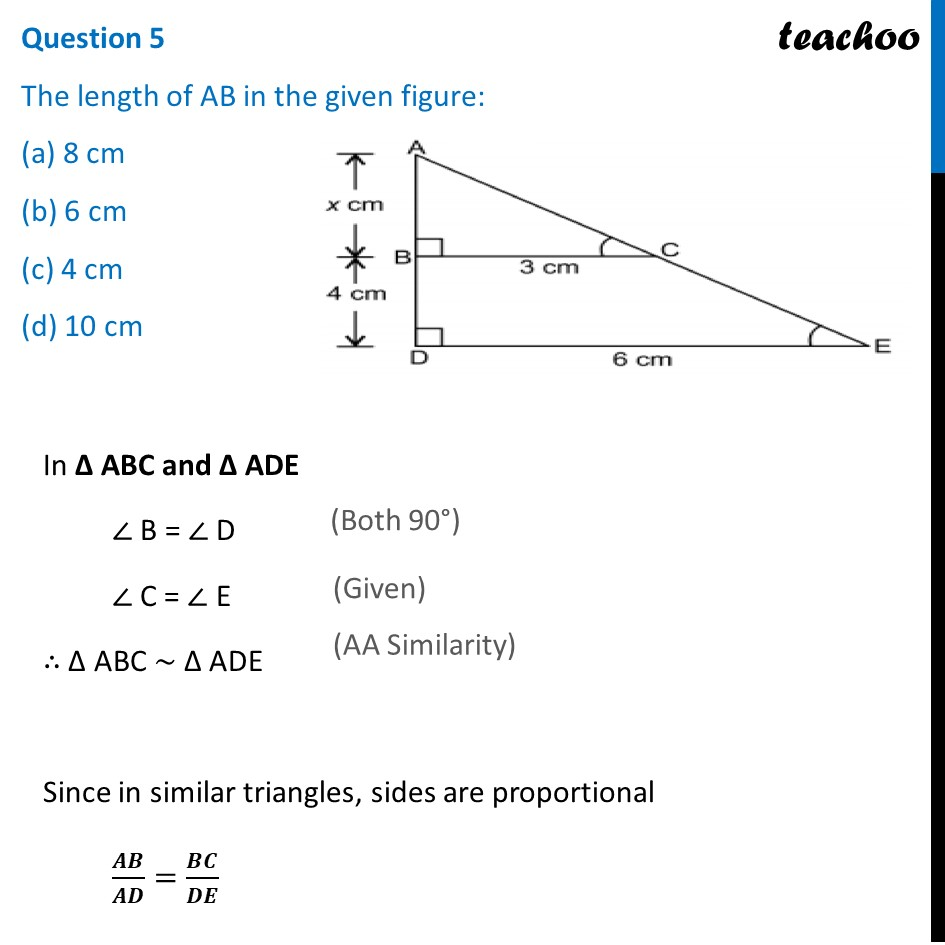1. Chapter 6 Class 10 Triangles (Term 1)
2. Serial order wise
3. Case Based Questions (MCQ)

Transcript

Question A scale drawing of an object is the same shape at the object but a different size. The scale of a drawing is a comparison of the length used on a drawing to the length it represents. The scale is written as a ratio. The ratio of two corresponding sides in similar figures is called the scale factor Scale factor = length in image / corresponding length in object If one shape can become another using revising, then the shapes are similar. Hence, two shapes are similar when one can become the other after a resize, flip, slide or turn. In the photograph below showing the side view of a train engine. Scale factor is 1:200This means that a length of 1 cm on the photograph above corresponds to a length of 200cm or 2 m, of the actual engine. The scale can also be written as the ratio of two lengths.Question 1 If the length of the model is 11cm, then the overall length of the engine in the photograph above, including the couplings(mechanism used to connect) is: (a) 22 cm (b) 220 cm (c) 220 m (d) 22 m(a) 22 cm Given that Scale factor is 1 : 200 Therefore, (𝑳𝒆𝒏𝒈𝒕𝒉 𝒐𝒇 𝒎𝒐𝒅𝒆𝒍 𝒆𝒏𝒈𝒊𝒏𝒆)/(𝑳𝒆𝒏𝒈𝒕𝒉 𝒐𝒇 𝒓𝒆𝒂𝒍 𝒍𝒊𝒇𝒆 𝒆𝒏𝒈𝒊𝒏𝒆)=𝟏/𝟐𝟎𝟎 (11 𝑐𝑚)/(𝐿𝑒𝑛𝑔𝑡ℎ 𝑜𝑓 𝑟𝑒𝑎𝑙 𝑙𝑖𝑓𝑒 𝑒𝑛𝑔𝑖𝑛𝑒)=1/200 11 × 200 cm = Length of Real life engine 2200 cm = Length of Real life engine Length of real life engine = 2200 cm Length of real life engine = 2200/100 cm Length of real life engine = 22 m So, the correct answer is (d) Question 2 What will affect the similarity of any two polygons? (a) They are flipped horizontally (b) They are dilated by a scale factor (c) They are translated down (d) They are not the mirror image of one another. Let’s check each option Option A – When they are flipped After flipping the size of polygons remain the same Since the two polygons are of the size ∴ They will remain similar Option B – They are dilated by a scale factor Since they are made smaller in size (dilated) by the same scale factor Both similar triangles become smaller, but ratio of their sides remains the same ∴ They will remain similar Option C – They are translated down This is similar to flipping. They are just moved around, but the size remains the same ∴ They will remain similar Option D – They are not mirror image of one another If they are not mirror image of one another, it means their size has changed. Since the size has changed, they do not remain similar. So, the correct answer is (d) Question 3 What is the actual width of the door if the width of the door in photograph is 0.35 cm? (a) 0.7 m (b) 0.7 cm (c) 0.07 cm (d) 0.07 m Given that Scale factor is 1 : 200 Therefore, (𝑾𝒊𝒅𝒕𝒉 𝒐𝒇 𝒅𝒐𝒐𝒓 𝒊𝒏 𝒑𝒉𝒐𝒕𝒐𝒈𝒓𝒂𝒑𝒉)/(𝑨𝒄𝒕𝒖𝒂𝒍 𝒘𝒊𝒅𝒕𝒉 𝒐𝒇 𝒕𝒉𝒆 𝒅𝒐𝒐𝒓)=𝟏/𝟐𝟎𝟎 (0.35 𝑐𝑚)/(𝑨𝒄𝒕𝒖𝒂𝒍 𝒘𝒊𝒅𝒕𝒉 𝒐𝒇 𝒕𝒉𝒆 𝒅𝒐𝒐𝒓)=1/200 0.35 × 200 cm = Actual width of the door 35/100 × 200 cm = Actual width of the door 35 × 2 cm = Actual width of the door 70 cm = Actual width of the door Actual width of the door = 70 cm Actual width of the door = 70/100 m Actual width of the door = 0.7 m So, the correct answer is (a) Question 4 If two similar triangles have a scale factor 5 : 3 which statement regarding the two triangles is true? (a) The ratio of their perimeters is 15 : 1 (b) Their altitudes have a ratio 25 : 15 (c) Their medians have a ratio 10 : 4 (d) Their angle bisectors have a ratio 11 : 5 When two triangles have ratio 5 : 3, then Medians have the same ratio – 5 : 3 Altitudes have the same ratio – 5 : 3 Perimeters have the same ratio – 5 : 3 Since 𝟐𝟓/𝟏𝟓=𝟓/𝟑, ∴ Their altitudes have a ratio 25 : 15 So, the correct answer is (b) Question 5 The length of AB in the given figure: (a) 8 cm (b) 6 cm (c) 4 cm (d) 10 cm In Δ ABC and Δ ADE ∠ B = ∠ D ∠ C = ∠ E ∴ Δ ABC ~ Δ ADE Since in similar triangles, sides are proportional 𝑨𝑩/𝑨𝑫=𝑩𝑪/𝑫𝑬 𝑥/(𝑥 + 4)=3/6 𝑥/(𝑥 + 4)=1/2 2x = x + 4 2x − x = 4 x = 4 cm So, the correct answer is (c)

Case Based Questions (MCQ)